# Eigenvalues, Eigenvectors of Tensors Civil Engineering (CE) Notes | EduRev

## Civil Engineering (CE) : Eigenvalues, Eigenvectors of Tensors Civil Engineering (CE) Notes | EduRev

The document Eigenvalues, Eigenvectors of Tensors Civil Engineering (CE) Notes | EduRev is a part of the Civil Engineering (CE) Course Advanced Solid Mechanics - Notes, Videos, MCQs & PPTs.
All you need of Civil Engineering (CE) at this link: Civil Engineering (CE)

Eigenvalues, eigenvectors of tensors
The scalar λi characterize eigenvalues (or principal values) of A if there exist corresponding nonzero normalized eigenvectors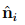(or principal directions or principal axes) of A, so that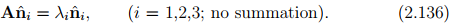To identify the eigenvectors of a tensor, we use subsequently a hat on the vector quantity concerned, for example,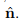.
Thus, a set of homogeneous algebraic equations for the unknown eigenvalues λi , i = 1,2,3, and the unknown eigenvectors, i = 1,2,3, is
(A − λi1)= o, (i = 1,2,3; no summation).                                            (2.137)

For the above system to have a solution≠ o the determinant of the system must vanish. Thus,

det(A − λi1) = 0                                                                                        (2.138)

where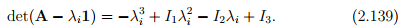This requires that we solve a cubic equation in λ, usually written as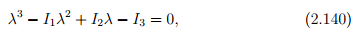called the characteristic polynomial (or equation) for A, the solutions of which are the eigenvalues λi , i = 1,2,3. Here, Ii , i = 1,2,3, are the so-called principal scalar invariants of A and are given by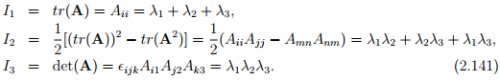If A is invertible then we can compute I2 using the expression I2 = tr(A−1 ) det(A). A repeated application of tensor A to equation (2.136) yields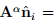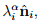i = 1,2,3, for any positive integer α. (If A is invertible then α can be any integer; not necessarily positive.) Using this relation and (2.140) multiplied by, we obtain the well-known Cayley-Hamilton equation: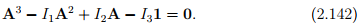It states that every second order tensor A satisfies its own characteristic equation. As a consequence of Cayley-Hamilton equation, we can express Aα in terms of A2 , A, and principal invariants for positive integer, α > 2. (If A is invertible, the above holds for any integer value of α positive or negative provided α ≠ {0, 1, 2}.)

For a symmetric tensor S the characteristic equation (2.140) always has three real solutions and the set of eigenvectors form a orthonormal basis {nˆi} (the proof of this statement is omitted). Hence, for a positive definite symmetric tensor A, all eigenvalues λi are (real and) positive since, using (2.136), we have λi =�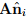> 0, i = 1,2,3.

Any symmetric tensor S may be represented by its eigenvalues λ, i = 1,2,3, and the corresponding eigenvectors of S forming an orthonormal basis {}. Thus, S can be expressed as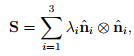(2.143)

called the spectral representation (or spectral decomposition) of S. Thus, when orthonormal eigenvectors are used as the Cartesian basis to represent S then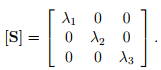(2.144)

The above holds when all the three eigenvalues are distinct. On the other hand, if there exists a pair of equal roots, i.e., λ1 = λ2 ≠  λ3, with an unique eigenvector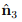associated with λ3, we deduce that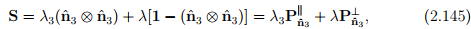where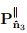and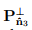denote projection tensors introduced in (2.109) and (2.110) respectively. Finally, if all the three eigenvalues are equal, i.e., λ1 = λ= λ3 = λ, then

S = λ1,                                                                              (2.146)

where every direction is a principal direction and every set of mutually orthogonal basis denotes principal axes.
It is important to recognize that eigenvalues characterize the physical nature of the tensor and that they do not depend on the coordinates chosen.
Square root theorem Let C be symmetric and positive definite tensor. Then there is a unique positive definite, symmetric tensor U such that

U2 = C.                            (2.147)

We write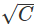for U. If spectral representation of C is: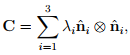(2.148)

then the spectral representation for U is: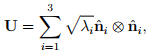(2.149)

where we have assumed that the eigenvalues of C, λare distinct. On the other hand if λ1 = λ2 = λ3 = λ then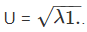(2.150)

If λ1 = λ2 = λ ≠ λ3, with an unique eigenvectorassociated with λ3 then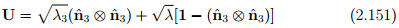Offer running on EduRev: Apply code STAYHOME200 to get INR 200 off on our premium plan EduRev Infinity!

## Advanced Solid Mechanics - Notes, Videos, MCQs & PPTs

42 videos|61 docs

,

,

,

,

,

,

,

,

,

,

,

,

,

,

,

,

,

,

,

,

,

,

,

,

;